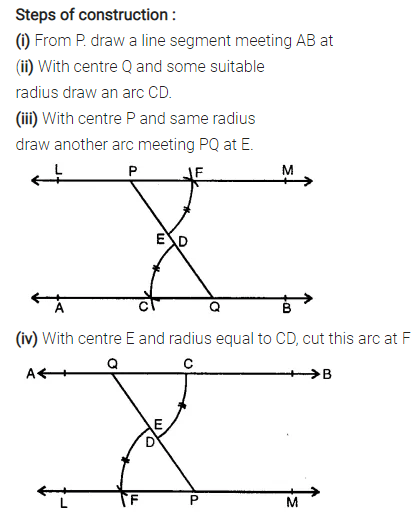# Selina Concise Mathematics Class 7 ICSE Solutions Chapter 14 Lines and Angles

## Selina Concise Mathematics Class 7 ICSE Solutions Chapter 14 Lines and Angles (Including Construction of angles)

Selina Publishers Concise Mathematics Class 7 ICSE Solutions Chapter 14 Lines and Angles (Including Construction of angles)

### Lines and Angles Exercise 14A – Selina Concise Mathematics Class 7 ICSE Solutions

Question 1.
State, true or false :
(i) A line segment 4 cm long can have only 2000 points in it.
(ii) A ray has one end point and a line segment has two end-points.
(iii) A line segment is the shortest distance between any two given points.
(iv) An infinite number of straight lines can be drawn through a given point.
(v) Write the number of end points in
(a) a line segment AB (b) arayAB
(c) a lineAB
(vi) Out of $$\overleftrightarrow { AB }$$ , $$\overrightarrow { AB }$$ , $$\overleftarrow { AB }$$ and $$\overline { AB }$$ , which one has a fixed length?
(vii) How many rays can be drawn through a fixed point O?
(viii) How many lines can be drawn through three
(a) collinear points?
(b) non-collinear points?
(ix) Is 40° the complement of 60°?
(x) Is 45° the supplement of 45°?
Solution: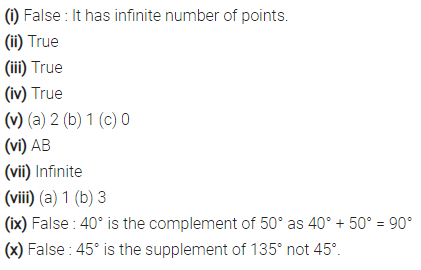Question 2.
In which of the following figures, are ∠AOB and ∠AOC adjacent angles? Give, in each case, reason for your answer.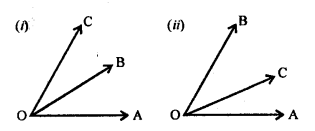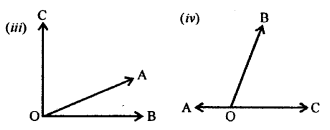Solution: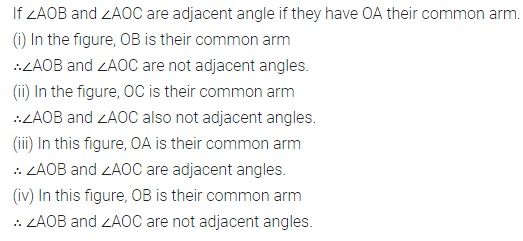Question 3.
In the given figure, B AC is a straight line.
Find : (i) x (ii) ∠AOB (iii) ∠BOC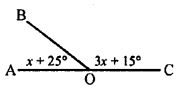Solution:Question 4.
Find yin the given figure.Solution:Question 5.
In the given figure, find ∠PQR.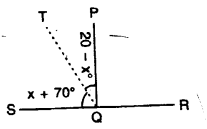Solution: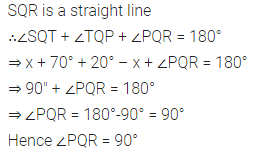Question 6.
In the given figure. p° = q° = r°, find each.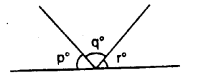Solution:Question 7.
In the given figure, if x = 2y, find x and y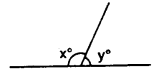Solution: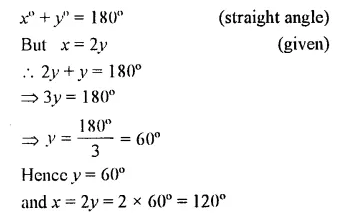Question 8.
In the adjoining figure, if b° = a° + c°, find b.Solution: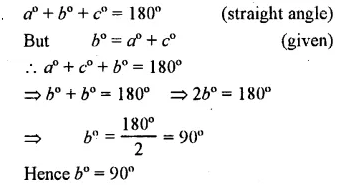Question 9.
In the given figure, AB is perpendicular to BC at B.
Find : (i) the value of x.
(ii) the complement of angle x.Solution:Question 10.
Write the complement of: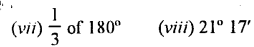Solution:Question 11.
Write the supplement of:
(i) 100°
(ii) 0°
(iii) x°
(iv) (x + 35)°
(v) (90 +a + b)° f
(vi) (110 – x – 2y)°
(vii) $$\frac { 1 }{ 5 }$$ of a right angle
(viii) 80° 49′ 25″
Solution: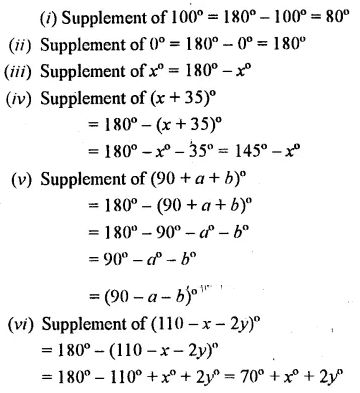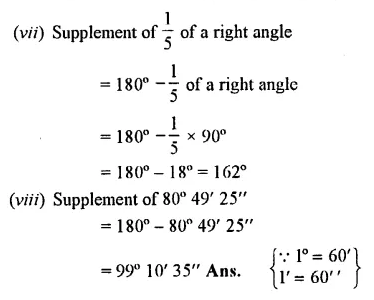Question 12.
Are the following pairs of angles complementary ?
(i) 10° and 80°
(ii) 37° 28′ and 52° 33′
(iii) (x+ 16)°and(74-x)°
(iv) 54° and $$\frac { 2 }{ 5 }$$ of a right angle.
Solution: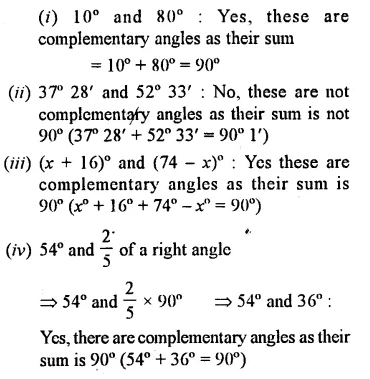Question 13.
Are the following pairs of angles supplementary?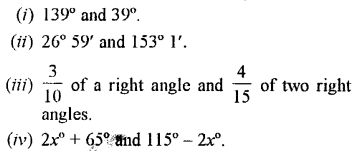Solution: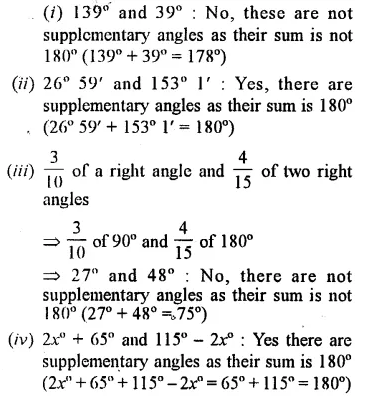Question 14.
If 3x + 18° and 2x + 25° are supplementary, find the value of x.
Solution:Question 15.
If two complementary angles are in the ratio 1:5, find them.
Solution:Question 16.
If two supplementary’ angles are in the ratio 2 : 7, find them.
Solution: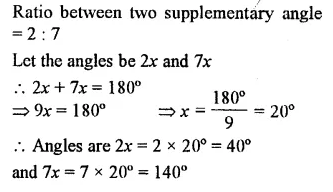Question 17.
Three angles which add upto 180° are in the ratio 2:3:7. Find them.
Solution:Question 18.
20% of an angle is the supplement of 60°. Find the angle.
Solution: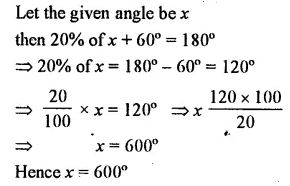Question 19.
10% of x° is the complement of 40% of 2x°. Find x
Solution: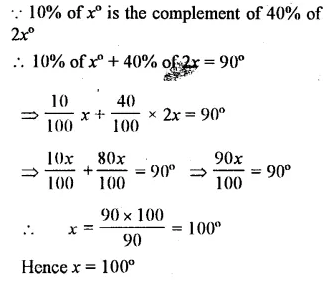Question 20.
Use the adjacent figure, to find angle x and its supplement.Solution:Question 21.
Find k in each of the given figures.Solution:Question 22.
In the given figure, lines PQ, MN and RS intersect at O. If x : y = 1 : 2 and z = 90°, find ∠ROM and ∠POR.Solution:Question 23.
In the given figure, find ∠AOB and ∠BOC.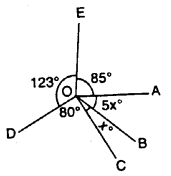Solution: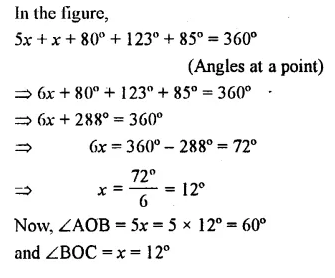Question 24.
Find each angle shown in the diagram.Solution: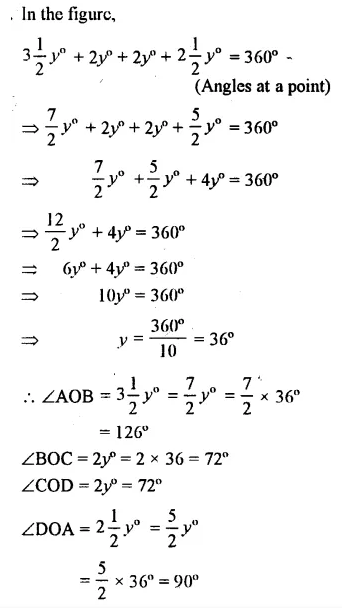Question 25.
AB, CD and EF are three lines intersecting at the same point.
(i) Find x, if y = 45° and z = 90°.
(ii) Find a, if x = 3a, y = 5x and r = 6x.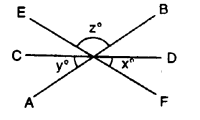Solution:### Lines and Angles Exercise 14B – Selina Concise Mathematics Class 7 ICSE Solutions

In questions 1 and 2, given below, identify the given pairs of angles as corresponding angles, interior alternate angles, exterior alternate angles, adjacent angles, vertically opposite angles or allied angles :
Question 1.
(i) ∠3 and ∠6
(ii) ∠2 and ∠4
(iii) ∠3 and ∠7
(iv) ∠2 and ∠7
(v) ∠4 and∠6
(vi) ∠1 and ∠8
(vii) ∠1 and ∠5
(viii) ∠1 and ∠4
(ix) ∠5 and ∠7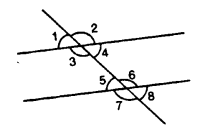Solution:Question 2.
(i) ∠1 and ∠4
(ii) ∠4 and ∠7
(iii) ∠10 and ∠12
(iv) ∠7 and ∠13
(v) ∠6 and ∠8
(vi) ∠11 and ∠8
(vii) ∠7 and ∠9
(viii) ∠4 and ∠5
(ix) ∠4 and ∠6
(x) ∠6 and ∠7
(xi) ∠2 and ∠13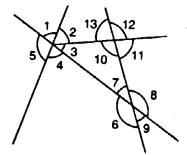Solution:Question 3.
In the given figures, the arrows indicate parallel lines. State which angles are equal. Give reasons.Solution:Question 4.
In the given figure, find the measure of the unknown angles :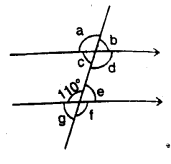Solution: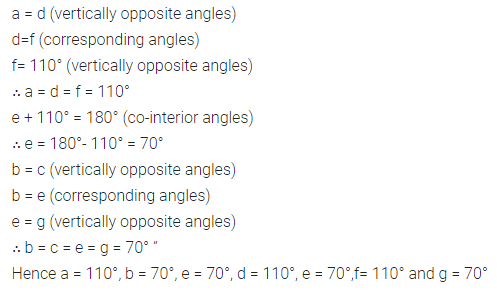Question 5.
Which pair of the dotted line, segments, in the following figures, are parallel. Give reason:Solution:Question 6.
In the given figures, the directed lines are parallel to each other. Find the unknown angles.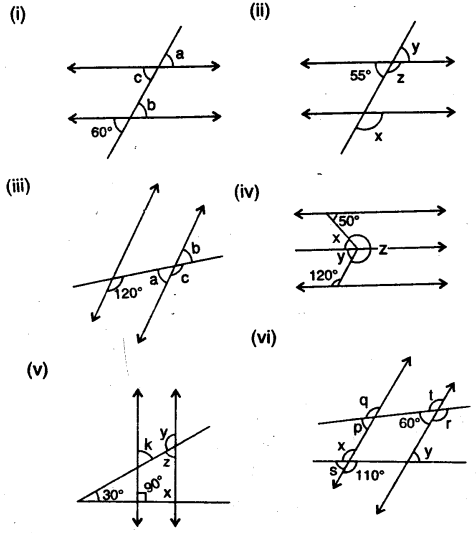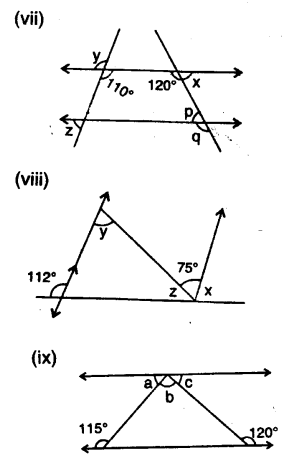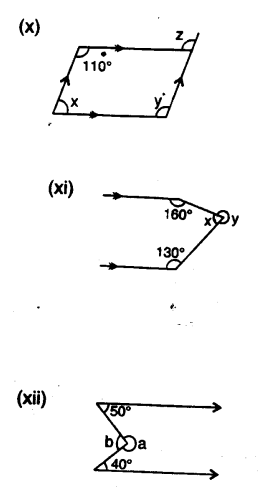Solution: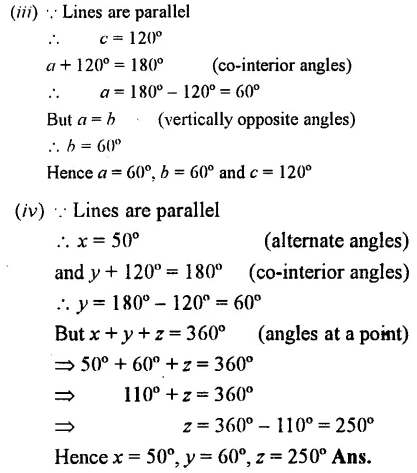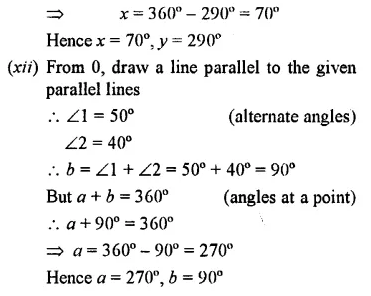>

Question 7.
Find x. y and p is the given figures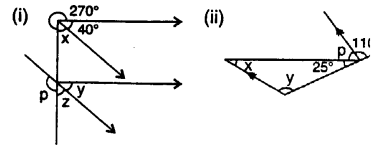Solution: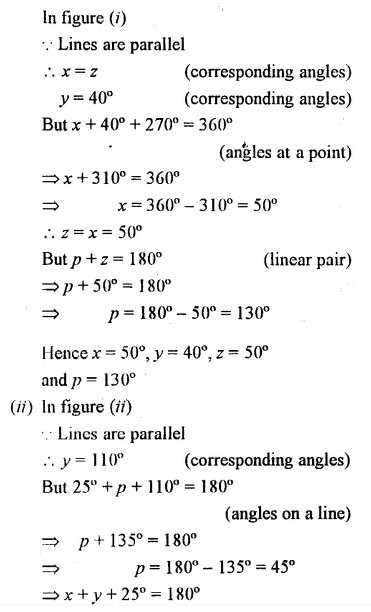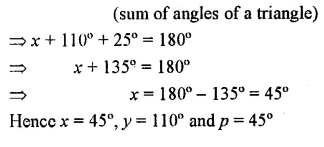Question 8.
Find x in the following cases :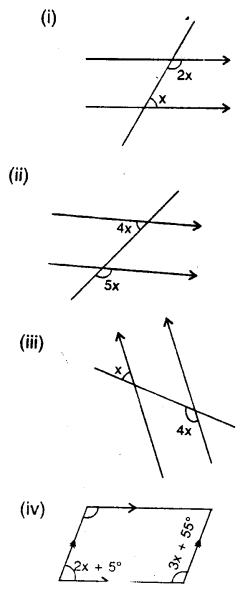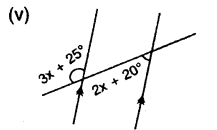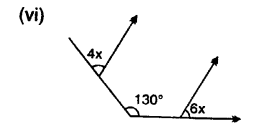Solution: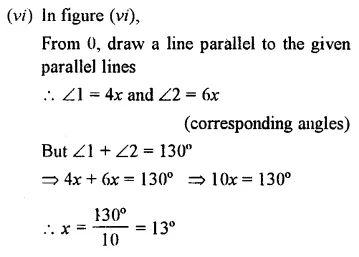### Lines and Angles Exercise 14C – Selina Concise Mathematics Class 7 ICSE Solutions

Question 1.
Using ruler and compasses, construct the following angles :
(i)30°
(ii)15°
(iii) 75°
(iv) 180°
(v) 165°
(vi) 22.5°
(vii) 37.5°
(viii) 67.5°
Solution: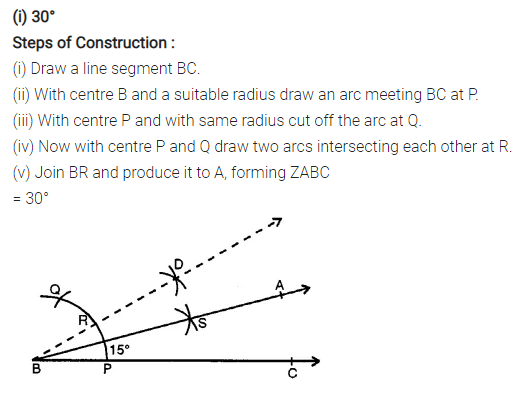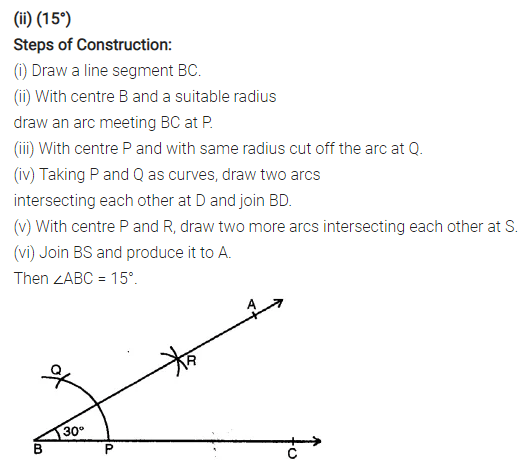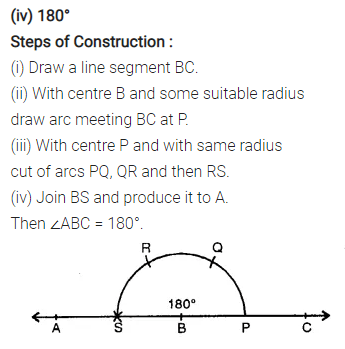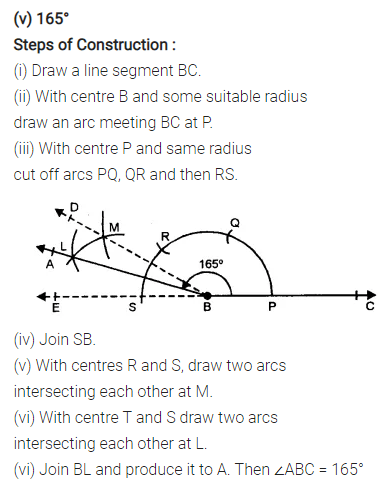Question 2.
Draw ∠ABC = 120°. Bisect the angle using ruler and compasses only. Measure each 1 angle so obtained and check whether the angles obtained on bisecting ∠ABC are equal or not.
Solution: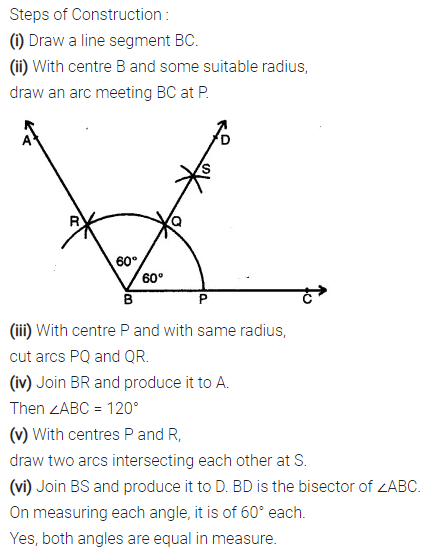Question 3.
Draw a line segment PQ = 6 cm. Mark a point A in PQ so that AP = 2 cm. At point A, construct angle QAR = 60°.
Solution:Question 4.
Draw a line segment AB = 8 cm. Mark a point P in AB so that AP = 5 cm. At P, construct angle APQ = 30°.
Solution:Question 5.
Construct an angle of 75° and then bisect it.
Solution:Question 6.
Draw a line segment of length 6 .4 cm. Draw its perpendicular bisector.
Solution: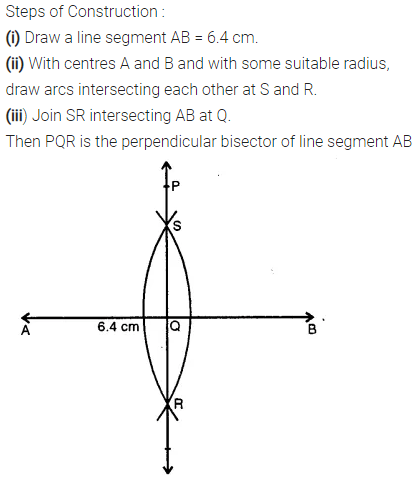Question 7.
Draw a line segment AB = 5.8 cm. Mark a point P in AB such that PB = 3.6 cm. At P, draw perpendicular to AB.
Solution: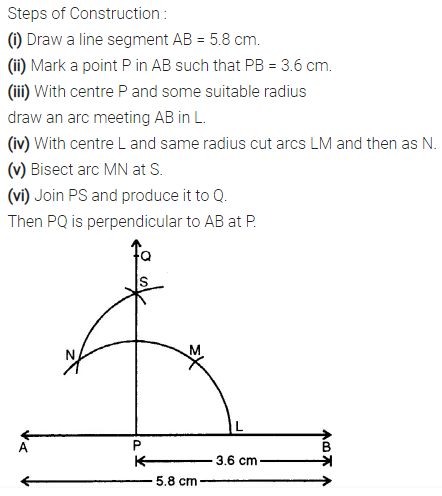Question 8.
In each case, given below, draw a line through point P and parallel to AB :Solution: